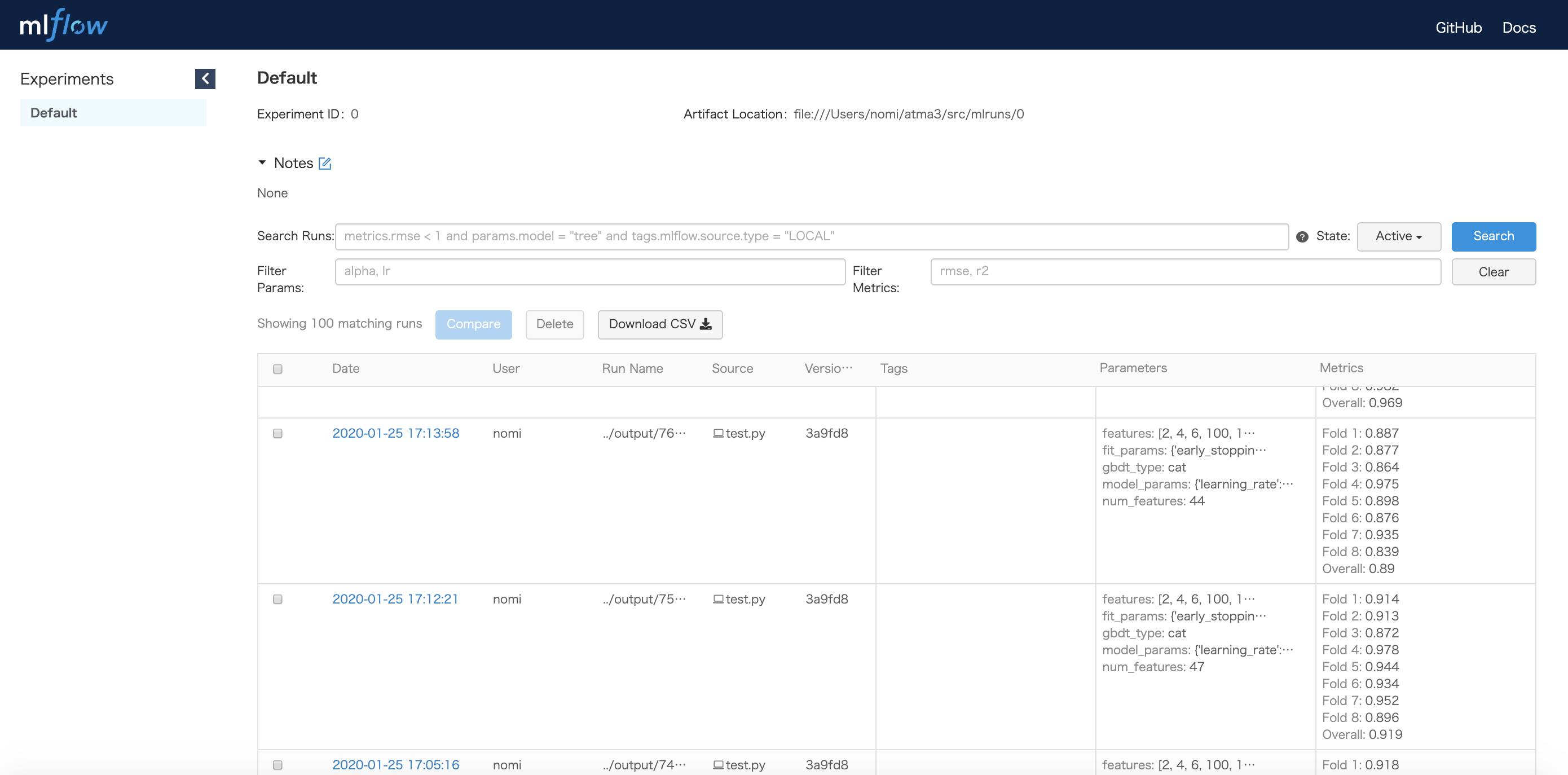# Tracking your machine learning experiments with run_experiment¶

## Concept¶

In a typical tabular data competition, you may probably repeat evaluating your idea by cross-validation with logging the parameters and results to track your experiments.

The `nyaggle.experiment.run_experiment` is an API for such situations. If you are using LightGBM as your model, the code will be quite simple:

```import pandas as pd
from nyaggle.experiment import run_experiment
from nyaggle.experiment import make_classification_df

INPUT_DIR = '../input'
target_column = 'target'

y = X_train[target_column]
X_train = X_train.drop(target_column, axis=1)

lightgbm_params = {
'max_depth': 8
}

result = run_experiment(lightgbm_params,
X_train,
y,
X_test,
sample_submission=sample_df)
```

The `run_experiment` API performs cross-validation and stores artifacts to the logging directory. You will see the output files stored as follows:

```output
└── 20200130123456          # yyyymmssHHMMSS
├── params.json         # Parameters
├── metrics.json        # Metrics (single fold & overall CV score)
├── oof_prediction.npy  # Out of fold prediction
├── test_prediction.npy # Test prediction
├── 20200130123456.csv  # Submission csv file
├── importances.png     # Feature importance plot
├── log.txt             # Log file
└── models              # The trained models for each fold
├── fold1
├── fold2
├── fold3
├── fold4
└── fold5
```

Hint

The default validation strategy is a 5-fold CV. You can change this behavior by passing `cv` parameter (see API reference in detail).

If you want to use XGBoost, CatBoost or other sklearn estimators, specify the type of algorithm:

```# CatBoost
catboost_params = {
'eval_metric': 'Logloss',
'loss_function': 'Logloss',
'depth': 8,
}
result = run_experiment(catboost_params,
X_train,
y,
X_test,
algorithm_type='cat')

# XGBoost
xgboost_params = {
'objective': 'reg:linear',
'max_depth': 8
}
result = run_experiment(xgboost_params,
X_train,
y,
X_test,
algorithm_type='xgb')

# sklearn estimator
from sklearn.linear_model import Ridge
rigde_params = {
'alpha': 1.0
}
result = run_experiment(rigde_params,
X_train,
y,
X_test,
algorithm_type=Ridge)
```

Hint

The parameter will be passed to the constructor of sklearn API (e.g. `LGBMClassifier`).

## Collaborating with mlflow¶

If you want GUI dashboard to manage your experiments, you can use `run_experiment` with mlflow by just setting `with_mlfow = True` (you need to install mlflow beforehand).

```result = run_experiment(params,
X_train,
y,
X_test,
with_mlflow=True)
```

In the same directory as the script executed, run

```mlflow ui
```

and view it at http://localhost:5000 . On this page, you can see the list of experiments with CV scores and parameters.If you want to customize the behavior of logging, you can call `run_experiment` in the context of mlflow run. If there is an active run, `run_experiment` will use the currently active run instead of creating a new one.

```mlflow.set_tracking_uri('gs://ok-i-want-to-use-gcs')

with mlflow.start_run(run_name='your-favorite-run-name'):
mlflow.log_param('something-you-want-to-log', 42)

result = run_experiment(params,
X_train,
y,
X_test,
with_mlflow=True)
```

## What does `run_experiment` not do?¶

`run_experiment` can be considered as a mere cross-validation API with logging functionality. Therefore, you have to choose model parameters and perform feature engineering yourself.